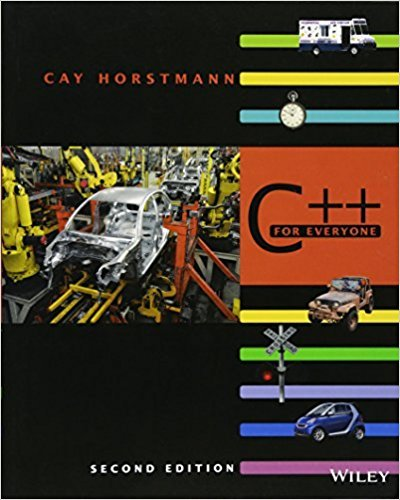×
Get Full Access to C++ For Everyone - 2 Edition - Chapter 5 - Problem R5.16
Get Full Access to C++ For Everyone - 2 Edition - Chapter 5 - Problem R5.16

×

# Solved: Consider the following function that is intended to swap the values of twoISBN: 9780470927137 356

## Solution for problem R5.16 Chapter 5

C++ for Everyone | 2nd Edition

• Textbook Solutions
• 2901 Step-by-step solutions solved by professors and subject experts
• Get 24/7 help from StudySoup virtual teaching assistantsC++ for Everyone | 2nd Edition

4 5 1 260 Reviews
24
3
Problem R5.16

Consider the following function that is intended to swap the values of two integers:void false_swap1(int& a, int& b){ a = b; b = a;}int main(){ int x = 3; int y = 4; false_swap1(x, y); cout << x << " " << y << endl; return 0;}Why doesnt the function swap the contents of x and y? How can you rewrite thefunction to work cor rectly?

Step-by-Step Solution:
Step 1 of 3

­last resort, use else statement. Cannot put anything after the “else” expression or it won’t read it ­only “if” and “end” are needed ­ the “elseif” and and “else” are not NEEDED >> %Day 7 MATLAB examples >> a=10;b=15;c=20; >> x=a> y=a>c||b> %one means yes, zero means no >> z=a>c&&b> %these are 3 logical operators >> v=x&y v = 1 >> %if x is true and y is true, you should get a true for v as well >> %string compare syntax is "strcmp('Yes','No') >> %ex. s1='upon';s2={'Once','Upon','A','Time'};tf=strcmp(s1,s2) >> s1='upon';s2={'Once','Upon','A','Time'};tf=strcmp(s1,s2) tf = 0 0 0 0 >> %It's comparing each thing and saying yes this is true (1) or no this is false (0) >> %example below >> strcmp('Test','test') ans = 0 >> %the above just asked MATLAB if capitalized test is different than lowercase test and it gave false because that is not true >> %examples with if­else statements ­there are 2 inputs we can use in our script: a string or a number ­if you put: strcmp(‘trig,’sine’) it compares your input named ‘trig’ to the sine function, and if you input sine, it will execute the command In class exercise: Answer: trig=input('Enter a trig function,'s​;%be sure to include the string indicator x=[0:pi/2:2*pi];%X values for the plot i strcmp(trig,'sine)%will run if sine is entered y=sin(x)%creates the y values for the plot plot(x,y%plots the

Step 2 of 3

Step 3 of 3

## Discover and learn what students are asking

Calculus: Early Transcendental Functions : The Natural Logarithmic Function: Integration
?In Exercises 1-26, find the indefinite integral. $$\int \frac{9}{5-4 x} d x$$

Calculus: Early Transcendental Functions : Introduction to Functions of Several Variables
?In Exercises 19 - 30, find the domain and range of the function. $$f(x, y)=x^{2}+y^{2}$$

Statistics: Informed Decisions Using Data : Organizing Qualitative Data
?In a relative frequency distribution, what should the relative frequencies add up to?

Statistics: Informed Decisions Using Data : Inference on the Least-Squares Regression Model and Multiple Regression
?A nutritionist wants to develop a model that describes the relation between the calories, total fat content, protein, sugar, and carbohydrates in chee

Statistics: Informed Decisions Using Data : Inference about the Difference between Two Medians: Dependent Samples
?Effects of Exercise A physical therapist wishes to learn whether an exercise program increases flexibility. She measures the flexibility (in inches) o

Unlock Textbook Solution